# Exploring the Open Access Evidence Base in Unpaywall with Python

Open Access evidence sources constantly change. In this blog post, I present a Python based approach for analysing the most recent snapshots from the open access discovery service Unpaywall. Results shows a growth in open access content, partly because of newly introduced evidence sources like Semantic Scholar.

Nick Haupka
March 30, 2020

Unpaywall makes millions of scholarly fulltexts from a variety of different repositories and services like PubMed Central and the Directory of Open Access Journals (DOAJ) discoverable. Although Unpaywall offers a REST API to query the Unpaywall database, sometimes it is more convenient and efficient to use database snapshots, which the Unpaywall team usually released twice a year.

In this blog post, I discuss the results from the former blog post “Open Access Evidence in Unpaywall” by comparing different database snapshots obtained from Unpaywall. Since the article´s publication on May 7th, 2019, Unpaywall has updated its data snapshots. Therefore, I want to explore potential changes in the Unpaywall data over time. For this post I used the most recent Unpaywall data dump from February 2020. Instead of using R, I present how to analyse the Unpaywall dump with Python.

The SUB scholarly communication analytics team regularly stores the Unpaywall snapshot on Google BigQuery. To query it in Python, I use the google-cloud-bigquery package. By default, this package does not ship with the data analysis tool pandas. However, I recommend to use this package along with pandas for a better experience, because the queried data can be represented as a pandas DataFrame.


import pandas as pd
from matplotlib import pyplot as plt
import matplotlib.patches as mpatches
import matplotlib.ticker as mtick
import seaborn as sns
import upsetplot

The setup for the Google BigQuery Python client is pretty straight forward. First, I import bigquery from the google.cloud package and create a client object by passing the project name as a parameter. If you did not set your credentials for the Google Cloud service yet, Google asks you to verify your client. This can be done by exporting an API-Key provided by Google into the working environment or by installing the official Google Cloud SDK. A detailed description on how to authenticate the client can be found here. Notice that the project-database has restricted access.


client = bigquery.Client(project='api-project-764811344545')

Like in the aforementioned blog post, also the recent dataset is stored in two tables, containing records between 2008 and February 2020. For the analysis, I will restrict the dataset being used here to publication years 2008 until 2019, the time period covered in the previous post plus the recent year 2019. For reusability, I define two variables which are holding the tables’ names to call them in SQL queries. I will also use the improved string formatting syntax which is a new feature since Python 3.6.


# database snapshots
upw_08_12 = 'oadoi_full.mongo_upwFeb20_08_12'
upw_13_19 = 'oadoi_full.mongo_upwFeb20_13_20'

I can query the project-database by using the query method on my created client object. In this example I requested ten journal articles that were published in 2019. I can pass the SQL query simply as a string into the query method. Next I can chain the to_dataframe method on my query to get a pandas DataFrame.


client.query(f"""
SELECT *
FROM {upw_13_19}
WHERE year=2019 AND genre="journal-article"
LIMIT 10
""").to_dataframe()
#>                         doi  ...  year
#> 0       10.3906/kim-1808-51  ...  2019
#> 1  10.3389/fimmu.2019.01664  ...  2019
#> 2  10.3847/1538-4357/ab464c  ...  2019
#> 3            10.5334/pb.443  ...  2019
#> 4         10.1063/1.5088767  ...  2019
#> 5  10.3389/fimmu.2019.02009  ...  2019
#> 6         10.1530/ey.16.7.4  ...  2019
#> 7       10.7554/elife.44364  ...  2019
#> 8        10.1242/bio.041467  ...  2019
#> 9      10.1051/cocv/2019042  ...  2019
#>
#> [10 rows x 14 columns]

## Paratexts in Unpaywall (is_paratext)

As can be seen from the outcome of the previous query, Unpaywall has recently introduced a new attribute is_paratext in the updated February 2020 database snapshot. It contains a boolean value which indicates whether a DOI is linked to a paratext or not. Because additional content to a journal article can also get classified as a journal article by the publisher, such as table of contents, it can mislead the analysis of scholarly articles in Unpaywall. Therefore I will ignore DOI´s that are related to paratexts in the following. For more information about the is_paratext field visit this page.

But firstly, I will analyze the share of paratexts in the current data dump. For that I request the total number of DOI´s and the number of DOI´s that are related to paratexts in both tables.


paratext_08_12 = client.query(f"""
SELECT
COUNT(nullif(is_paratext = true, false))
AS number_of_paratexts,
COUNT(doi)
AS number_of_all_dois,
FROM (
SELECT DISTINCT(doi), is_paratext, genre
FROM {upw_08_12}
WHERE genre="journal-article"
)
""").to_dataframe()

paratext_13_19 = client.query(f"""
SELECT
COUNT(nullif(is_paratext = true, false))
AS number_of_paratexts,
COUNT(doi)
AS number_of_all_dois,
FROM (
SELECT DISTINCT(doi), is_paratext, year, genre
FROM {upw_13_19}
WHERE year<2020 AND genre="journal-article"
)
""").to_dataframe()

paratext_df = pd.concat([paratext_08_12, paratext_13_19])
paratext_df = paratext_df.sum().to_frame().transpose()
paratext_df = paratext_df.eval('prop = (number_of_paratexts/number_of_all_dois) * 100')
paratext_df.prop = paratext_df.prop.apply(lambda x: '{0:.2f}'.format(x))
paratext_df.columns = ['Number of DOI´s identified as paratext',
'Number of all DOI´s',
'Proportion of all Paratexts in %']

paratext_df
Table 1: Number of paratexts in Unpaywall.
Number of DOI´s identified as paratext Number of all DOI´s Proportion of all Paratexts in %
321385 35875237 0.90

Yet, the proportion of paratexts in the dataset amounts to approximately 1%. In total, 321385 paratexts are linked to a specific DOI. You can also see that 35,875,237 distinct DOIs of genre journal-article are included in the excerpt of the current database snapshot, containing publications from 2008 onwards.

## Open Access availability (is_oa)

To contrast the previous results from the blog post with the recent results, I begin with a comparison of the total number of articles between the two datasets from February 2019 and February 2020. More importantly, I will investigate the open access share between these two.

Before I can compute the open access proportion in the dataset, I must query the database and count the number of distinct DOI´s by year and open access status. Since I have two tables, I need to concatenate the dataframes in the next step. I also have to convert the column which contains the year from string to datetime. This allows me to handle date information much better when visualizing data. After I have calculated the proportion of open access by year, I will now sort the values by time.


oa_08_12 = client.query(f"""
SELECT year, is_oa, COUNT(DISTINCT(doi)) AS n
FROM {upw_08_12}
WHERE genre="journal-article" AND is_paratext=False
GROUP BY year, is_oa
""").to_dataframe()

oa_13_19 = client.query(f"""
SELECT year, is_oa, COUNT(DISTINCT(doi)) AS n
FROM {upw_13_19}
WHERE year<2020 AND genre="journal-article" AND is_paratext=False
GROUP BY year, is_oa
""").to_dataframe()

df = pd.concat([oa_08_12, oa_13_19])
df.year = pd.to_datetime(df.year.apply(lambda x: str(x) + "-01-01"))
df['prop'] = df.groupby(['year'])['n'].transform(lambda x: x / x.sum())
df = df.sort_values(by=['year', 'is_oa'], ascending=[True, False]).reset_index(drop=True)
#>         year  is_oa        n      prop
#> 0 2008-01-01   True   616069  0.299712
#> 1 2008-01-01  False  1439469  0.700288
#> 2 2009-01-01   True   697145  0.310508
#> 3 2009-01-01  False  1548033  0.689492
#> 4 2010-01-01   True   789565  0.317344

For visualization, I will use matplotlib. Matplotlib is a commonly used plotting library for Python that is influenced by Matlab and enables a wide range of different plot figures. By using matplotlib I can also take advantage of pandas plotting methods which uses the library as the standard backend.

In the first plot I visualize the open access share to journal articles over time. Whereas the plot is not interactive like in the stated blog post, if needed, this could be achieved by using the same plotting library named Plotly which can be found here. Plotly is available for R, Javascript and Python.


plt.style.use('seaborn-whitegrid')

ax = df.groupby(['year', 'is_oa'], sort=False) \
['n'].sum().unstack() \
.plot.area(figsize=(10,4),
alpha=0.8,
xlim=(37.5, 49.5),
linewidth=0,
color=['#56B4E9', '#b3b3b3a0'])

ax.grid(False, which='both', axis='x')
handles, labels = ax.get_legend_handles_labels()
plt.box(False)

plt.xlabel('Year published', labelpad=10, fontdict={'fontsize': 11, 'fontweight': 500})
plt.ylabel('Journal Articles', labelpad=10, fontdict={'fontsize': 11, 'fontweight': 500})

plt.legend(handles, ['TRUE', 'FALSE'],
title='Is OA?',
fontsize='medium',
bbox_to_anchor=(1.15, 1.02),
labelspacing=1.2)

plt.tight_layout()

plt.show()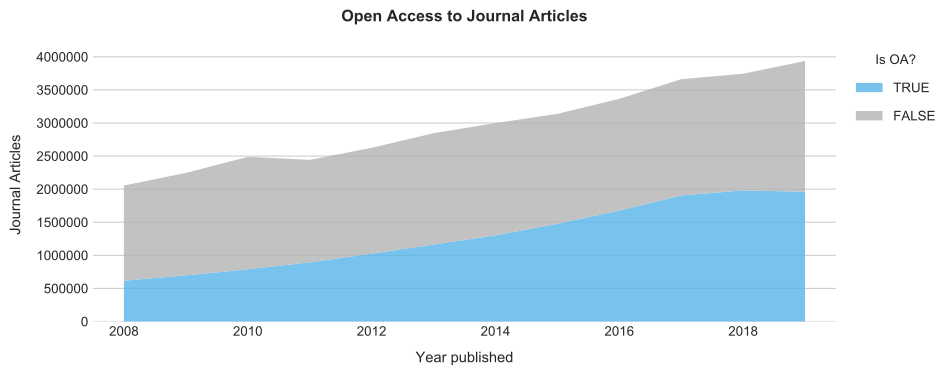As expected, the total number of journal articles has increased, compared to the previous results from the blog post. In fact, the number of articles with a distinct DOI included in the Unpaywall data dump from February 2020 has increased by 14% (regardless of paratexts that were not specified in previous database snapshots) in comparison to the findings from the original blog post. In addition, the share of open access articles has increased by 7%. Overall, 15,487,801 articles that were published between 2008 and 2019 are freely available by February 2020.

## Unpaywall Open Access Hosting Types (host_type)

Next, I investigate the differences between the distribution of the host types specified in the data dumps. The host type describes the type of location that serves open access full-texts and accepts two values: publisher and repository. As mentioned in the blog post, the host type variable is determined by Unpaywall’s algorithm.


HOST_TYPE_08_12_QUERY = f"""
SELECT year, host_type, journal_is_in_doaj,
COUNT(DISTINCT(doi)) AS number_of_articles
FROM {upw_08_12}, UNNEST (oa_locations)
WHERE genre="journal-article" AND is_best=true AND is_paratext=False
GROUP BY year, host_type, journal_is_in_doaj
"""

HOST_TYPE_13_19_QUERY = f"""
SELECT year, host_type, journal_is_in_doaj,
COUNT(DISTINCT(doi)) AS number_of_articles
FROM {upw_13_19}, UNNEST (oa_locations)
WHERE genre="journal-article" AND year<2020 AND is_best=true
AND is_paratext=False
GROUP BY year, host_type, journal_is_in_doaj
"""

Like in the original blog post, I create a host column with the pandas provided loc method to highlight freely available full-texts provided by DOAJ-indexed journals in addition to the regular host types. Because the DOAJ has comprehensive standards for journal inclusion, it might be interesting to see whether an increase of publishing in potentially less strict open access journals can be observed or not.


host_type_08_12_query_df = client.query(HOST_TYPE_08_12_QUERY).to_dataframe()
host_type_13_19_query_df = client.query(HOST_TYPE_13_19_QUERY).to_dataframe()

host_type_df = pd.concat([host_type_08_12_query_df, host_type_13_19_query_df])
host_type_df.year = pd.to_datetime(host_type_df.year.apply(lambda x: str(x) + "-01-01"))
host_type_df = host_type_df.sort_values(by=['year']).reset_index(drop=True)

host_type_df.loc[host_type_df['host_type'] == 'publisher', 'host'] = 'Other Journals'
host_type_df.loc[host_type_df['host_type'] == 'repository', 'host'] = 'Repositories only'
host_type_df.loc[host_type_df['journal_is_in_doaj'] == True, 'host'] = 'DOAJ-listed Journal'

#>         year   host_type  ...  number_of_articles                 host
#> 0 2008-01-01   publisher  ...              309659       Other Journals
#> 1 2008-01-01  repository  ...              213820    Repositories only
#> 2 2008-01-01   publisher  ...               92590  DOAJ-listed Journal
#> 3 2009-01-01   publisher  ...              349556       Other Journals
#> 4 2009-01-01  repository  ...              232924    Repositories only
#>
#> [5 rows x 5 columns]

Again, I visualize the data with matplotlib. In contrast to the R package ggplot2, I found it a bit more inconvenient to prepare and plot data with matplotlib in Python. This starts by iterating over the host types to generate a subplot for each. Although I can make use of pandas plotting methods to display complicated graphics from DataFrames, it requires much effort to get publication quality figures.


all_articles = host_type_df.groupby(['year'])['number_of_articles'].sum() \
.reset_index(name='number_of_articles')

x = all_articles['year'].dt.year
y_total = all_articles.number_of_articles

plt.style.use('seaborn-whitegrid')

fig, axes = plt.subplots(nrows=1, ncols=3, figsize=(12,3.8))

fontsize=14,
fontweight=600,
y=1.10)

for i, host in enumerate(host_type_df.host.unique(), 1):

ax = plt.subplot(1,3,i)

y_stacked = host_type_df[host_type_df.host==host] \
.groupby(['year'])['number_of_articles'].sum() \
.reset_index(name='number_of_articles').number_of_articles

ax.bar(x, y_total, color='#b3b3b3a0', label='All OA Articles')
ax.bar(x, y_stacked, color='#56B4E9', label='by Host')

ax.set_title(host, fontdict={'fontsize': 12, 'fontweight': 500})

ax.set_frame_on(False)
ax.grid(False, which='both', axis='x')
ax.set(xlabel='', ylabel='')

if i > 1:
ax.set_yticklabels([], visible=False)

# common xlabel
fig.text(0.45, -0.03, 'Year', ha='center',
fontdict={'fontsize': 12, 'fontweight': 500})

# common ylabel
fig.text(-0.02, 0.5, 'OA Articles (Total)', va='center', rotation='vertical',
fontdict={'fontsize': 12, 'fontweight': 500})

plt.legend(fontsize='medium', bbox_to_anchor=(1.05, 0.9), labelspacing=1.2)

fig.tight_layout()

plt.show()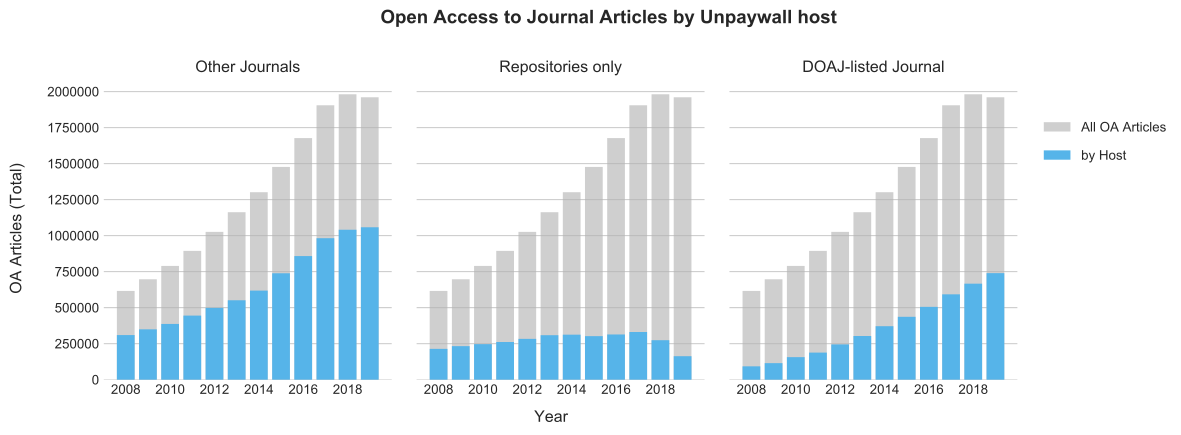Figure 2: Open access to journal articles by open access hosting location.

The figure highlights that the proportion of open access articles provided by journals which are not listed in DOAJ rapidly increased in the year 2018 in comparison to the previous results. Nonetheless, the overall share of articles obtained from journals that are not listed in DOAJ decreased from 56% to 51%. Consequently, the share of open access articles provided by DOAJ-listed journals increased.

## Unpaywall Open Access Evidence Types (evidence)

In the following, I explore varieties between the evidence types of the two data dumps. The evidence type is a variable determined by Unpaywall, which expresses the location at which an article was found and how Unpaywall was able to identify the open access status of an article.


EVIDENCE_08_12_QUERY = f"""
SELECT evidence, year, is_best,
COUNT(DISTINCT(doi)) AS number_of_articles
FROM {upw_08_12}, UNNEST (oa_locations)
WHERE genre="journal-article" AND is_paratext=False
GROUP BY evidence, year, is_best
"""

EVIDENCE_13_19_QUERY = f"""
SELECT evidence, year, is_best,
COUNT(DISTINCT(doi)) AS number_of_articles
FROM {upw_13_19}, UNNEST (oa_locations)
WHERE genre="journal-article" AND year < 2020 AND is_paratext=False
GROUP BY evidence, year, is_best
"""

evidence_08_12 = client.query(EVIDENCE_08_12_QUERY).to_dataframe()
evidence_13_19 = client.query(EVIDENCE_13_19_QUERY).to_dataframe()

evidence_df = pd.concat([evidence_08_12, evidence_13_19])
evidence_df.year = pd.to_datetime(evidence_df.year.apply(lambda x: str(x) + "-01-01"))

#>                                   evidence  ... number_of_articles
#> 0  oa repository (semantic scholar lookup)  ...              54888
#> 1  oa repository (semantic scholar lookup)  ...              74108
#> 2         oa repository (via pmcid lookup)  ...               5397
#> 3  oa repository (semantic scholar lookup)  ...              64668
#> 4  oa repository (semantic scholar lookup)  ...              56898
#>
#> [5 rows x 4 columns]

For each evidence type I calculate the total number of articles and the related proportion, as well as the cumulative proportion with respect to the number of all articles. Then I display the results in form of a table. To specify the precision of the resulting floating point numbers I again make use of the Python built-in string formatting function. Here, I specify by 2 digits of accuracy.


articles_per_type_df = evidence_df.groupby(['evidence']).number_of_articles \
.sum() \
.to_frame() \
.reset_index() \
.sort_values(by=['number_of_articles'], ascending=False) \
.reset_index(drop=True)

articles_per_type_df['prop'] = articles_per_type_df['number_of_articles'] \
.transform(lambda x:
x / articles_per_type_df['number_of_articles'] \
.sum() * 100)

articles_per_type_df['cumul'] = articles_per_type_df.prop.cumsum()

articles_per_type_table = articles_per_type_df.copy()
articles_per_type_table.prop = articles_per_type_table.prop \
.apply(lambda x: '{0:.2f}'.format(x))

articles_per_type_table.cumul = articles_per_type_table.cumul \
.apply(lambda x: '{0:.2f}'.format(x))

articles_per_type_table.columns = ['Evidence Types',
'Number of Articles',
'Proportion of all Articles in %',
'Cumulative Proportion in %']

articles_per_type_table
Table 2: Number of articles per evidence type.
Evidence Types Number of Articles Proportion of all Articles in % Cumulative Proportion in %
0 open (via free pdf) 5342059 16.33 16.33
1 oa repository (via OAI-PMH doi match) 5069032 15.50 31.83
2 open (via page says license) 4948332 15.13 46.96
3 oa repository (semantic scholar lookup) 4621741 14.13 61.09
4 oa journal (via doaj) 4320534 13.21 74.30
5 oa repository (via pmcid lookup) 3704354 11.33 85.63
6 oa repository (via OAI-PMH title and first author match) 1950563 5.96 91.59
7 oa journal (via observed oa rate) 1508233 4.61 96.20
8 open (via crossref license) 614330 1.88 98.08
9 open (via free article) 265035 0.81 98.89
10 open (via page says Open Access) 95838 0.29 99.18
11 oa journal (via publisher name) 77084 0.24 99.42
12 open (via crossref license, author manuscript) 75537 0.23 99.65
13 oa repository (via OAI-PMH title match) 67689 0.21 99.86
14 oa repository (via OAI-PMH title and last author match) 46905 0.14 100.00
15 manual 29 0.00 100.00
16 hybrid (via page says license) 1 0.00 100.00

Interestingly, the evidence type oa repository (semantic scholar lookup) ranked fourth was not included in the database snapshot used in the May 2019 analysis. Further, the same phenomenon as in the previous work can be observed: the least frequent eight evidence types summarized only make up 1.9% of all articles in total. In the following, I will collate these evidence types in the category other.


list_of_small_evidence_types = articles_per_type_df \
.loc[articles_per_type_df['prop'] < 1] \
.evidence.tolist()

articles_per_type_grouped_df = articles_per_type_df.copy()

articles_per_type_grouped_df.evidence = articles_per_type_grouped_df \
.evidence.replace(list_of_small_evidence_types, 'other')

articles_per_type_grouped_df = articles_per_type_grouped_df \
.groupby(['evidence']) \
.number_of_articles.sum() \
.to_frame().reset_index() \
.sort_values(by=['number_of_articles'], ascending=False) \
.reset_index(drop=True)

articles_per_type_grouped_df['prop'] = articles_per_type_grouped_df['number_of_articles'] \
.transform(lambda x:
x / articles_per_type_grouped_df['number_of_articles'].sum() * 100)

articles_per_type_grouped_df['cumul'] = articles_per_type_grouped_df.prop.cumsum()

evidence_grouped_df = evidence_df.copy()

evidence_grouped_df.evidence = evidence_grouped_df \
.evidence.replace(list_of_small_evidence_types, 'other')

evidence_grouped_df = evidence_grouped_df.groupby(['evidence', 'is_best', 'year']) \
.number_of_articles.sum() \
.to_frame().reset_index() \
.sort_values(by=['number_of_articles'], ascending=False) \
.reset_index(drop=True)

To illustrate the best open access locations according to Unpaywall, I will visualize the quantity of articles that were obtained from data sources with the is_best attribute given by Unpaywall in contrast to the total number of articles found in each evidence type.

This time I will use the seaborn package for generating bar plots. Seaborn is a visualization library which is build on top of matplotlib. It is well designed to work with pandas DataFrames and it also enables smoother plots. Also, I can continue to use matplotlib methods.


evidence_grouped_plot_df = evidence_grouped_df[evidence_grouped_df.is_best == True] \
.groupby(['evidence'])['number_of_articles'] \
.sum().to_frame() \
.reset_index() \
.rename(columns={'number_of_articles': 'is_best_sum'})

evidence_grouped_plot_df = pd.merge(articles_per_type_grouped_df,
evidence_grouped_plot_df,
how='left',
on='evidence')

plt.style.use('seaborn-whitegrid')
fig = plt.figure(figsize=(7,5))
plt.box(False)

ax1 = sns.barplot('number_of_articles',
'evidence',
data=evidence_grouped_plot_df,
label='FALSE',
color='#b3b3b3a0',
alpha=0.6,
saturation=1)

ax2 = sns.barplot('is_best_sum',
'evidence',
data=evidence_grouped_plot_df,
label='TRUE',
color='#56B4E9',
alpha=1,
saturation=1)

plt.title('Number of Open Access Articles per Unpaywall Evidence Type',
fontdict={'fontsize': 12, 'fontweight': 600}, pad=20, x=0.3)
plt.xlabel('Number of Open Access Articles', labelpad=10,
fontdict={'fontsize': 11, 'fontweight': 500})

fontdict={'fontsize': 11, 'fontweight': 500})

plt.legend(title='Is best?', fontsize='medium', bbox_to_anchor=(1.2, 1.05), labelspacing=1.2)

plt.show()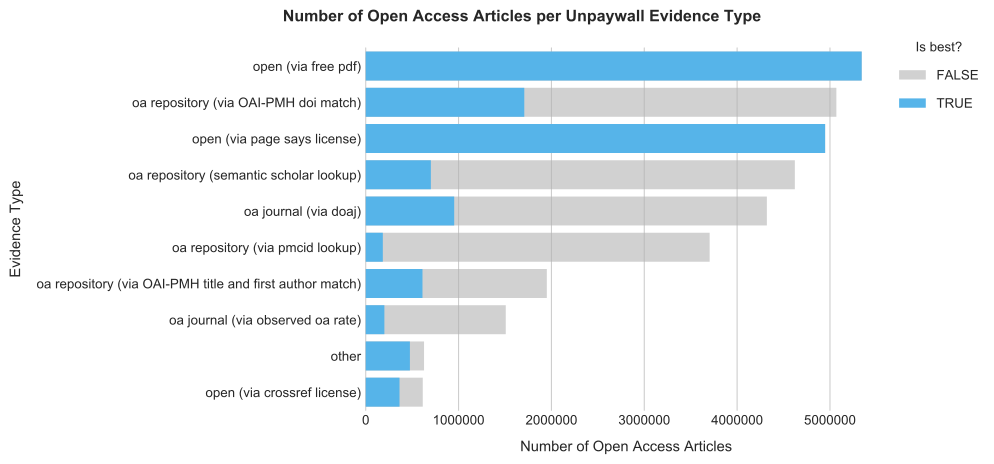Figure 3: Number of articles per evidence type.

The figure provides two types of evidence that were not specifically mentioned in the previous work: oa repository (semantic scholar lookup) and oa journal (via observed rate). Apparently, these evidence types are responsible for the overall increase in articles between the two data dumps from February 2019 and February 2020. Furthermore an increase of articles in repositories can be observed. Indeed, the evidence type with the second most associated articles is referring to repository locations. However, Unpaywall still prioritises publisher hosted content over repository depositions.

To distinguish the classification as best open access location of data sources by Unpaywall over time, I again make use of matplotlib. Although the FacetGrid function provided by seaborn would be very useful for this task it unfortunately doesn´t support stacked plots as mentioned in this issue on GitHub.


x = evidence_grouped_df.sort_values(by=['year'], ascending=True) \
.reset_index(drop=False) \
['year'].dt.year.unique()

plt.style.use('seaborn-whitegrid')

fig, axes = plt.subplots(nrows=5,
ncols=2,
sharex=True,
sharey=True,
figsize=(12,12))

# get a one-dimensional array
axes = axes.reshape(-1)

fig.suptitle('Unpaywall Open Access Evidence Categories per Year',
fontsize=15,
fontweight=600,
x=0.53,
y=1.04)

for i, ax in enumerate(axes, 1):

ax = plt.subplot(5,2,i, sharey=axes, sharex=axes)

if i % 2 == 0:
plt.setp(ax.get_yticklabels(), visible=False)

evidence = evidence_grouped_df.evidence.unique()[i-1]

y_total = evidence_grouped_df[evidence_grouped_df.evidence == evidence] \
.groupby(['year']) \
.number_of_articles.sum() \
.to_frame().reset_index() \
.number_of_articles

y_stacked = evidence_grouped_df[evidence_grouped_df.evidence == evidence] \
.loc[evidence_grouped_df.is_best == True] \
.groupby(['year']) \
.number_of_articles.sum() \
.to_frame().reset_index() \
.number_of_articles

ax = plt.bar(x, y_total, color='#b3b3b3a0')
ax = plt.bar(x, y_stacked, color='#56B4E9')
plt.title(evidence, fontdict={'fontsize': 12, 'fontweight': 500}, pad=0.2)

plt.grid(False, which='both', axis='x')
plt.box(False)

# common xlabel
fig.text(0.52, -0.03, 'Publication Year', ha='center',
fontdict={'fontsize': 13, 'fontweight': 500})

# common ylabel
fig.text(-0.04, 0.5, 'Number of Open Access Articles', va='center', rotation='vertical',
fontdict={'fontsize': 13, 'fontweight': 500})

is_best_patch = mpatches.Patch(color='#56B4E9', label='TRUE')
is_not_best_patch = mpatches.Patch(color='#b3b3b3a0', label='FALSE')
fig.legend(handles=[is_best_patch, is_not_best_patch], title='Is best?',
fontsize='large', title_fontsize='x-large',
bbox_to_anchor=(1.15, 0.95), labelspacing=1.2)

plt.tight_layout()

plt.show()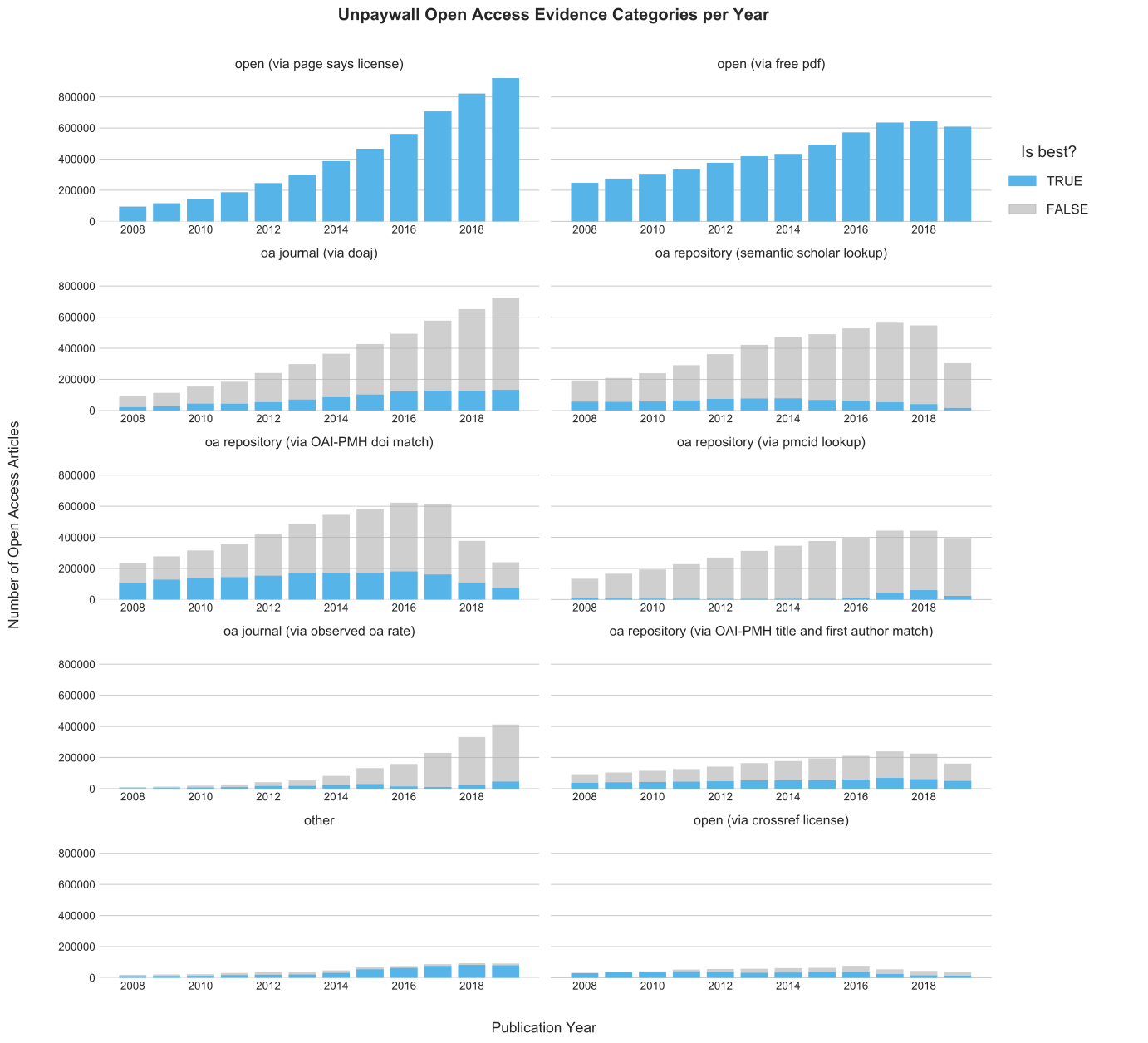Figure 4: Development of the number of articles per evidence type over time.

## Overlap of Open Access Provision and Evidence Types

Owing to possible multiple associations between an article and evidence types in Unpaywall (there might be multiple free access locations for the same article), I investigate the intersection between host types in the next step. Again, I compare the findings with the previous results.

### Overlap between Host Types


HOST_TYPE_INTERSECT_08_12_QUERY = f"""
SELECT year, host_type_count,
COUNT(DISTINCT(doi)) AS number_of_articles
FROM
(SELECT doi, year,
STRING_AGG(DISTINCT(host_type)
ORDER BY host_type) AS host_type_count
FROM {upw_08_12}, UNNEST (oa_locations)
WHERE genre="journal-article" AND is_paratext=False
GROUP BY doi, year)
GROUP BY host_type_count, year
ORDER BY number_of_articles DESC
"""

HOST_TYPE_INTERSECT_13_19_QUERY = f"""
SELECT year, host_type_count,
COUNT(DISTINCT(doi)) AS number_of_articles
FROM
(SELECT doi, year,
STRING_AGG(DISTINCT(host_type)
ORDER BY host_type) AS host_type_count
FROM {upw_13_19}, UNNEST (oa_locations)
WHERE genre="journal-article" AND year < 2020
AND is_paratext=False
GROUP BY doi, year)
GROUP BY host_type_count, year
ORDER BY number_of_articles DESC
"""

I start by querying the project-database and setting up the dataframe. Then I will merge the outcome with the articles_total_by_year dataframe which I have created before. This enables me to investigate the relative share of articles provided by different host types to the total number of articles across host types.


host_type_08_12_intersect_df = client.query(HOST_TYPE_INTERSECT_08_12_QUERY).to_dataframe()
host_type_13_19_intersect_df = client.query(HOST_TYPE_INTERSECT_13_19_QUERY).to_dataframe()
host_type_intersect_df = pd.concat([host_type_08_12_intersect_df,
host_type_13_19_intersect_df])

host_type_intersect_df.year = pd.to_datetime(host_type_intersect_df.year \
.apply(lambda x: str(x) + "-01-01"))

host_type_intersect_df \
.loc[host_type_intersect_df['host_type_count'] == 'publisher', 'host'] = 'Publisher only'
host_type_intersect_df \
.loc[host_type_intersect_df['host_type_count'] == 'publisher,repository',
'host'] = 'Publisher & Repository'
host_type_intersect_df \
.loc[host_type_intersect_df['host_type_count'] == 'repository',
'host'] = 'Repositories only'

articles_total_by_year_df = df.groupby(['year']).n.sum().to_frame().reset_index()
articles_total_by_year_df.columns = ['year', 'all_articles']

host_type_intersect_df = pd.merge(articles_total_by_year_df,
host_type_intersect_df, on='year', how='right')

host_type_intersect_df = host_type_intersect_df.groupby(['year', 'host']) \
.sum().eval('prop = number_of_articles/all_articles') \
.reset_index()

#>         year                    host  ...  number_of_articles      prop
#> 0 2008-01-01  Publisher & Repository  ...              187109  0.091027
#> 1 2008-01-01          Publisher only  ...              215140  0.104664
#> 2 2008-01-01       Repositories only  ...              213820  0.104021
#> 3 2009-01-01  Publisher & Repository  ...              221294  0.098564
#> 4 2009-01-01          Publisher only  ...              242927  0.108199
#>
#> [5 rows x 5 columns]

host_type_all = host_type_intersect_df.copy()
host_type_all['prop'] = host_type_intersect_df.groupby(['year'])['prop'] \
.transform(lambda x: x.sum())

Again, I will use seaborn to display the results.


# convert year column to work properly with seaborn
x = host_type_intersect_df['year'].dt.year

plt.style.use('seaborn-whitegrid')

fig, axes = plt.subplots(nrows=1, ncols=3, figsize=(11, 3.5))

fig.suptitle('Overlap between Open Access Host Types in Unpaywall',
fontsize=14,
fontweight=600,
x=0.48,
y=1.10)

for i, host in enumerate(host_type_intersect_df.host.unique(), 1):
ax = plt.subplot(1,3,i)
y_stacked = host_type_intersect_df[host_type_intersect_df.host == host].prop
sns.barplot(x,
'prop',
data=host_type_all,
color='#b3b3b3a0',
label='All OA Articles',
alpha=0.6,
saturation=1,
ci=None)

sns.barplot(x,
y_stacked,
color='#56B4E9',
label='by Host',
alpha=1,
saturation=1,
ci=None)

ax.set_title(host, fontdict={'fontsize': 12, 'fontweight': 500})

ax.set_frame_on(False)
ax.grid(False, which='both', axis='x')
ax.set(xlabel='', ylabel='')

# for readability I hide every second tick on the x axis
for label in ax.get_xticklabels()[1::2]:
label.set_visible(False)

ax.yaxis.set_major_formatter(mtick.PercentFormatter(1.0))

if i > 1:
ax.set_yticklabels([], visible=False)

# common xlabel
fig.text(0.45, -0.03, 'Year', ha='center',
fontdict={'fontsize': 12, 'fontweight': 500})

# common ylabel
fig.text(-0.02, 0.5, 'OA Share', va='center', rotation='vertical',
fontdict={'fontsize': 12, 'fontweight': 500})

plt.legend(bbox_to_anchor=(1.05, 0.9), labelspacing=1.2)

plt.tight_layout()

plt.show()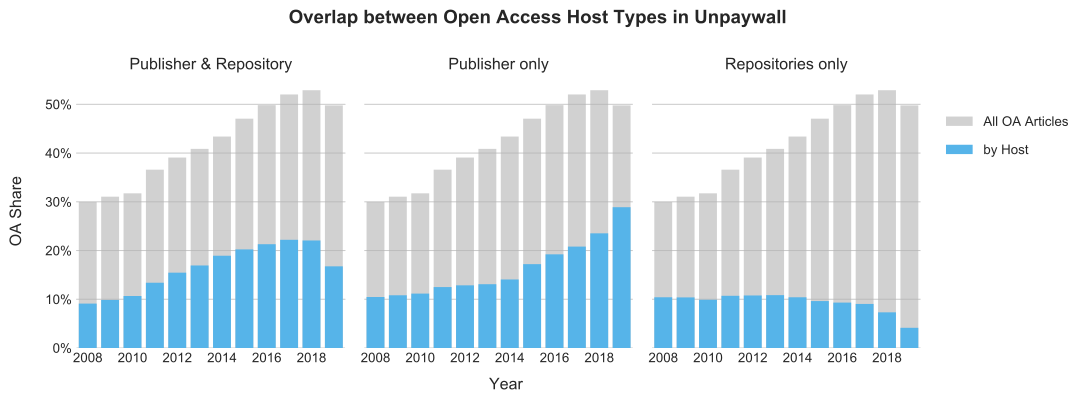Figure 5: Open access to journal articles by open access hosting location.

The figure shows that, in terms of percentage, fewer articles were found on publisher websites than in the previous blog post. Indeed, 79% of all open access full-texts are available through publisher websites which is a total of 12,246,005 articles. Relatively speaking, this is a decrease by 3% compared to the previous results. Also, the proportion of articles that are not archived in a repository has declined from 56% to 40%. Consequently, the proportion of articles that are available from both the publisher websites, as well as those that are archived in a repository have increased from 26% to 39%.

### Overlaps between Evidence types

So far, I investigated the overlap of host types in Unpaywall in this section. Next, I am going to analyze evidence types. Various articles are associated with multiple evidence types in Unpaywall as mentioned at the beginning of this section, so I examine the intersection between evidence types in the next step.


EVIDENCE_SINGLE_CAT_08_12_QUERY = f"""
SELECT ev_cat, COUNT(DISTINCT(doi)) AS number_of_articles
FROM
(SELECT doi, STRING_AGG(DISTINCT(evidence), "&"
ORDER BY evidence) AS ev_cat
FROM {upw_08_12}, UNNEST (oa_locations)
WHERE genre="journal-article" AND is_paratext=False
GROUP BY doi
)
GROUP BY ev_cat
"""

EVIDENCE_SINGLE_CAT_13_19_QUERY = f"""
SELECT ev_cat, COUNT(DISTINCT(doi)) AS number_of_articles
FROM
(SELECT doi, STRING_AGG(DISTINCT(evidence), "&"
ORDER BY evidence) AS ev_cat
FROM {upw_13_19}, UNNEST (oa_locations)
WHERE genre="journal-article" AND year < 2020
AND is_paratext=False
GROUP BY doi
)
GROUP BY ev_cat
"""

evidence_categories_08_12_df = client.query(EVIDENCE_SINGLE_CAT_08_12_QUERY).to_dataframe()
evidence_categories_13_19_df = client.query(EVIDENCE_SINGLE_CAT_13_19_QUERY).to_dataframe()
evidence_categories_df = pd.concat([evidence_categories_08_12_df,
evidence_categories_13_19_df])

evidence_categories_df = evidence_categories_df.groupby(['ev_cat']) \
.sum().reset_index() \
.sort_values(by=['number_of_articles'], ascending=False) \
.reset_index(drop=True)

#>                                               ev_cat  number_of_articles
#> 0                                open (via free pdf)             2476980
#> 1              oa repository (via OAI-PMH doi match)              771505
#> 2  oa journal (via doaj)&open (via page says lice...              734679
#> 3  oa repository (semantic scholar lookup)&open (...              726074
#> 4            oa repository (semantic scholar lookup)              665390

Again, I merge the dataframe with a previously created dataframe called evidence_df. Then I compute the frequency of open access full-texts related to a single data source. The result can be contrasted with the total number of articles found in each evidence type.


evidence_single_cat_df = evidence_df.groupby(['evidence']) \
.number_of_articles.sum() \
.reset_index()

evidence_single_cat_df = pd.merge(evidence_single_cat_df, evidence_categories_df,
how='left', left_on=['evidence'], right_on=['ev_cat']) \
.drop(['ev_cat'], axis=1)
evidence_single_cat_df.columns = ['evidence', 'number_of_articles', 'number_of_single_cat']

evidence_single_cat_df.evidence = evidence_single_cat_df \
.evidence.replace(list_of_small_evidence_types, 'other')

evidence_single_cat_grouped_df = evidence_single_cat_df.groupby(['evidence']) \
.sum() \
.eval('prop = number_of_single_cat/number_of_articles') \
.reset_index() \
.sort_values(by=['number_of_articles'], ascending=False) \
.reset_index(drop=True)

evidence_single_cat_grouped_df['number_of_single_cat'] = evidence_single_cat_grouped_df \
.number_of_single_cat \
.astype(int)

evidence_single_cat_grouped_df
#>                                             evidence  ...      prop
#> 0                                open (via free pdf)  ...  0.463675
#> 1              oa repository (via OAI-PMH doi match)  ...  0.152200
#> 2                       open (via page says license)  ...  0.131558
#> 3            oa repository (semantic scholar lookup)  ...  0.143970
#> 4                              oa journal (via doaj)  ...  0.105752
#> 5                   oa repository (via pmcid lookup)  ...  0.038285
#> 6  oa repository (via OAI-PMH title and first aut...  ...  0.247134
#> 7                  oa journal (via observed oa rate)  ...  0.108509
#> 8                                              other  ...  0.509406
#> 9                        open (via crossref license)  ...  0.412148
#>
#> [10 rows x 4 columns]

Next, I visualize the result in a horizontal barplot. For each evidence type I display the unique occurrences across related articles.


fig, axes = plt.subplots(figsize=(7,5))

plt.style.use('seaborn-whitegrid')
plt.box(False)

ax1 = sns.barplot(x= * len(evidence_single_cat_grouped_df),
y='evidence',
data=evidence_single_cat_grouped_df,
label='FALSE',
color='#b3b3b3a0',
saturation=1,
alpha=0.6
)

ax2 = sns.barplot(x='prop',
y='evidence',
data=evidence_single_cat_grouped_df,
label='TRUE',
color='#56B4E9',
saturation=1,
alpha=1
)

axes.xaxis.set_major_formatter(mtick.PercentFormatter(1.0))

plt.title('Proportion of Articles per Evidence Type',
fontdict={'fontsize': 12, 'fontweight': 600}, x=0.25, pad=15)
plt.show()